# A Guide to Using Linear Variable Filters (LVFs) in Spectrometers

This article describes how the spectral performance of the linear variable dichroic shown by FTLi750c.fsy depends on the width of the light spot (Figure 1) and angular distribution of the light incident on the dichroic filter.

The Linear variable dichroic filter from DELTA measures 60 mm in length and has a coated area length of 58.1 mm.

The edge is placed at 300 at one end of the dichroic and at nm 750 nm at the other end of it. The light spot is assumed to be defined by a rectangular slit 10 mms high and centered at 60% of the total length.

## Dependence on the Width of the Light-spot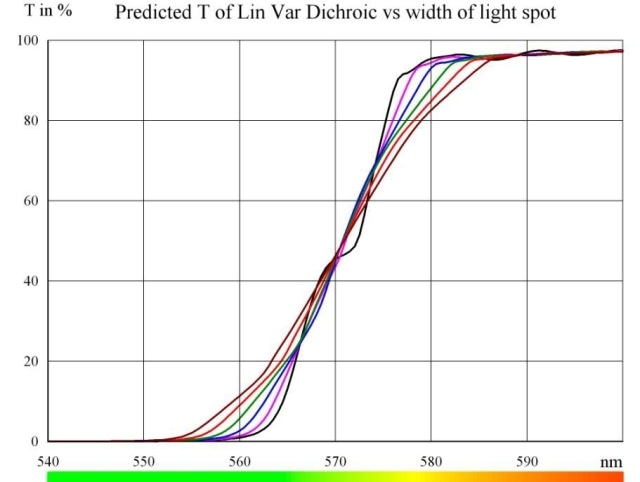Figure 1. Predicted transmission of unpolarized and parallel light at AOI = 45°. Black curve: width = 0.5 mm, violet curve: width = 1.0 mm, blue curve: width = 1.5 mm, green curve: width = 2.0 mm, red curve: width = 2.5 mm, brown curve: width = 3.0 mm.

With growing width of the light spot, the steepness of the edge reduces marginally. Based on the precise application, it might be possible to work with a width of a couple of millimeters or more.

## Dependence on the Angle of Incidence (AOI)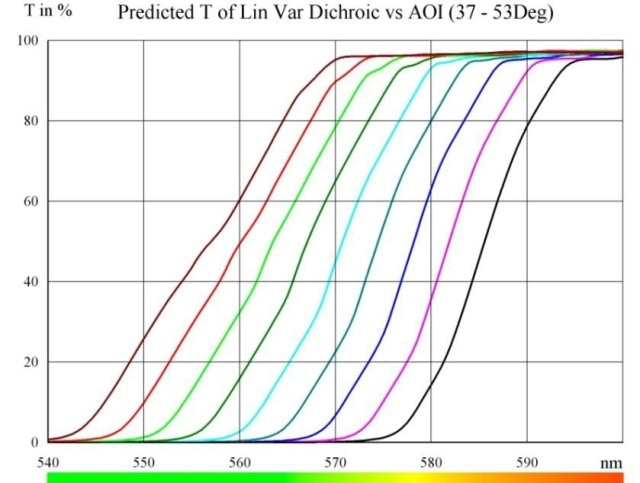Figure 2. Transmission curve shifts towards shorter wavelength as AOI increases

As the Angle of Incidence (AOI) increases, the transmission curve tends to move towards shorter wavelength (Figure 2). However, the shape of the edge is fairly stable in the angular range analyzed. AOI slowly increases from 45 – 8°. = 37° to 45 + 8°. = 53° in steps of 2°. The light spot width is maintained constant at 1.5 mm and the light is said to be unpolarized.

## Dependence on the Opening Angle (OA)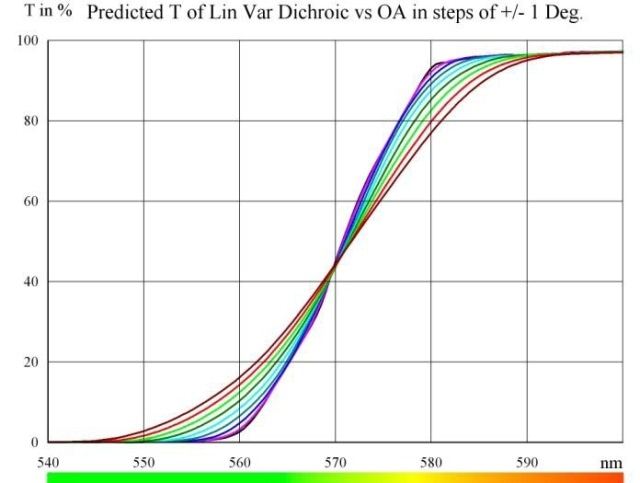3a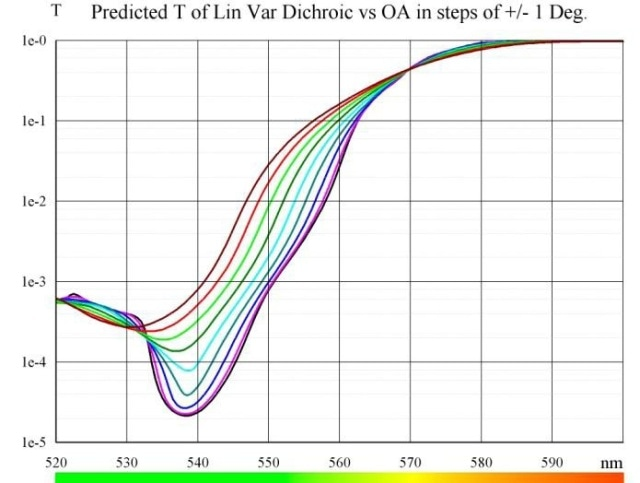3b

Figure 3a and 3b. Assuming an even angular energy distribution, in a non-parallel light bundle with an opening angle of OA°, the averaged transmission is predicted to be as shown

Assuming an even angular energy distribution, in a non-parallel light bundle with an opening angle of OA°, the averaged transmission is projected to be as depicted in Figures 3a and 3b. OA i.e. half cone angle is increasing from 0° to 8° in steps of 1°. Steepness of the subsequent edge reduces gradually with rising OA.

## Angling of Linear Variable Filters

It is common to tilt the filter marginally to prevent inter-reflections between filters positioned in series, and also to prevent direct reflection into a laser. Figure 4 shows a typical illustration of the angular dependence of a linear variable edge filter.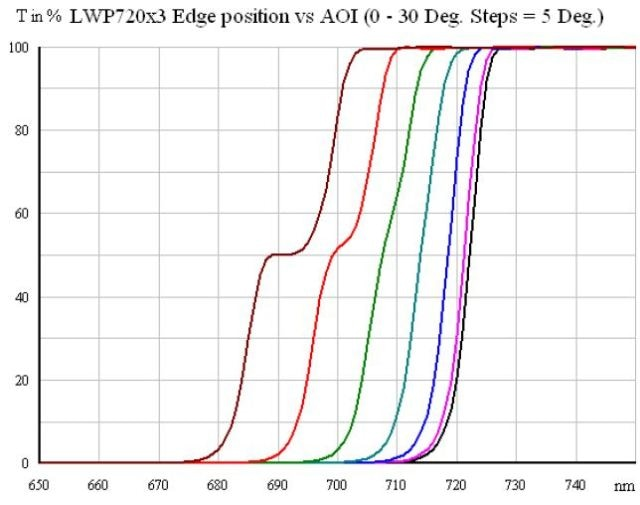Figure 4. LWP720x3 Edge position vs AOI (0 - 30°. Steps = 5°)

In the multi-layered coating, the phase thickness of the layers is illustrated by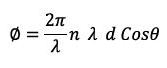Where n is the refractive index of the layer, ø is the wavelength of light, d is the physical thickness of the layer, and λ is the angle of light propagating in the layer.

According to Snells law, the angle of light in the layer is connected to the angle of incidence in air, V.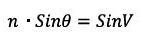Hence,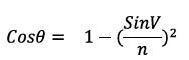The coating mainly has a vast number of layers, displaying a higher refractive index than the other every second. However, it is normal to add an effective refractive index to represent the whole multilayer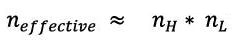As shown in Figure 4, the coating treated is composed of Ta2O5 and SiO2. At 720 nm wavelength, these materials have refractive indices of around 2.1085 and 1.4771 causing an actual refractive index of 1.7648.

By considering a wavelength of 721.5 nm at normal incidence (the T=50% point and the black curve), it is possible to calculate the values for the rest of the angles as shown in Figure 4.

• AOI= 5° => 720.6 nm
• AOI = 10° => 718.0 nm
• AOI = 15° => 713.7 nm
• AOI = 20° => 707.8 nm
• AOI = 25° => 700.5 nm
• AOI = 30° => 691.9 nm

While the values fit the curves quite well, polarization effects obviously course a severe distortion of the edge at angles of incidence of more than 20°. It is suggested to maintain the angles of incidence below 15°.

## Orientation of DELTA’s Linear Variable Filters

The paragraphs below explain how to orientate DELTA’s linear variable filters (LVFs) to make them perform exceptionally well.

### Orientation of LVFs with Respect to the Incident Light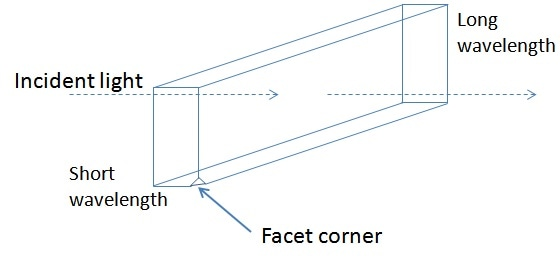Figure 5. Orientation of the filter with respect to the incident light

The filter substrate has a corner with a facet. It must be ensured that the light enters the filter from the opposite side, as shown in Figure 5. Incorrect mounting of the filter may lead to slight leakage of light in the blocking bands. The facet also marks the short wavelength end of the filter facet.

### Orientation of LVFs When Used in Series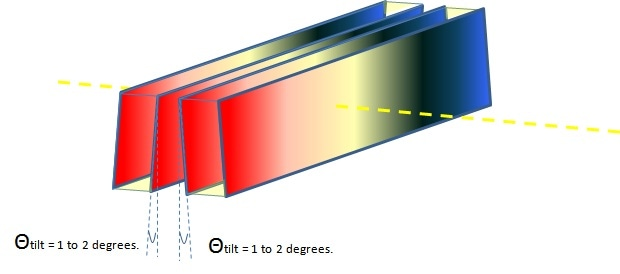Figure 6. Orientation of two LVFs when they are placed in series

When multiple LVFs are positioned in series either to build a bandpass with an LWP and an SWP or to enhance blocking with two similar filters, it is essential to decrease the inter reflections. This is done by tilting each filter a little with regard to the adjacent filters. It is advised to angle each filter by 1° to 2° in the vertical plan (Figure 6).

## Conclusion

DELTA’s LVFs are best suited for applications in spectroscopy. The spectral performance of the linear variable dichroic relies on the light spot’s width and angular distribution of the light incident on the dichroic filter. Orientation of DELTA’s LVFs is suggested to make them perform as much as possible.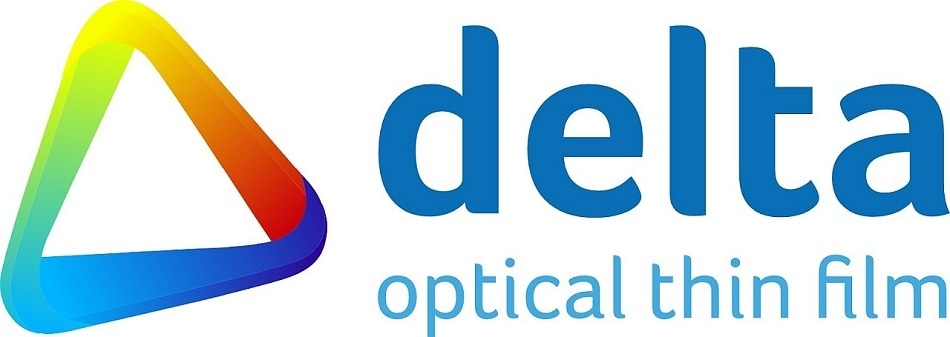This information has been sourced, reviewed and adapted from materials provided by DELTA Optical Thin Film.

## Citations

• APA

DELTA Optical Thin Film. (2019, July 10). A Guide to Using Linear Variable Filters (LVFs) in Spectrometers. AZoM. Retrieved on February 18, 2020 from https://www.azom.com/article.aspx?ArticleID=13780.

• MLA

DELTA Optical Thin Film. "A Guide to Using Linear Variable Filters (LVFs) in Spectrometers". AZoM. 18 February 2020. <https://www.azom.com/article.aspx?ArticleID=13780>.

• Chicago

DELTA Optical Thin Film. "A Guide to Using Linear Variable Filters (LVFs) in Spectrometers". AZoM. https://www.azom.com/article.aspx?ArticleID=13780. (accessed February 18, 2020).

• Harvard

DELTA Optical Thin Film. 2019. A Guide to Using Linear Variable Filters (LVFs) in Spectrometers. AZoM, viewed 18 February 2020, https://www.azom.com/article.aspx?ArticleID=13780.